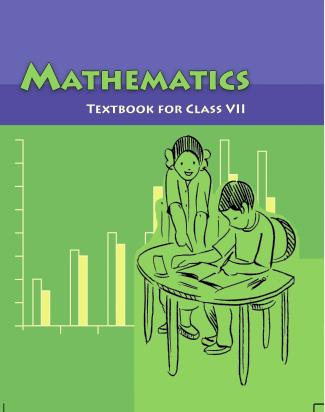1. /
2. CBSE
3. /
4. Class 07
5. /
6. Mathematics
7. /
8. NCERT Solutions for Class...

# NCERT Solutions for Class 7 Maths Exercise 3.2### myCBSEguide App

Download the app to get CBSE Sample Papers 2023-24, NCERT Solutions (Revised), Most Important Questions, Previous Year Question Bank, Mock Tests, and Detailed Notes.

NCERT solutions for Maths Data Handling## NCERT Solutions for Class 7 Maths Data Handling

###### Question 1.The scores in mathematics test (out of 25) of students is as follows:

19, 25, 23, 20, 9, 20, 15, 10, 5, 16, 25, 20, 24, 12, 20

Find the mode and median of this data. Are they same?

Arranging the given data in ascending order,

5, 9, 10, 12, 15, 16, 19, 20, 20, 20, 20, 23, 24, 25, 25

Mode is the observation occurred the highest number of times.

Therefore, Mode = 20

Median is the middle observation = 20

Yes, Mode and Median are same of given observation.

###### Question 2.The runs scored in a cricket match by 11 players is as follows:

6, 15, 120, 50, 100, 80, 10, 15, 8, 10, 15

Find the mean, mode and median of this data. Are the three same?

Arranging the given data in ascending order,

6, 8, 10, 10, 15, 15, 15, 50, 80, 100, 120

Mean = {tex}\frac{{{\text{Sum of observations}}}}{{{\text{Number of observations}}}}{/tex}

= {tex}\frac{{6 + 8 + 10 + 10 + 15 + 15 + 15 + 50 + 80 + 100 + 120}}{{11}}{/tex} = {tex}\frac{{429}}{{11}}{/tex} = 39

Mode is the observation occurred the highest number of times = 15

Median is the middle observation = 15

Therefore, Mode and Median is 15.

No, the mean, median and mode are not same.

NCERT Solutions for Class 7 Maths Exercise 3.2

###### Question 3.The weight (in kg) of 15 students of a class are:

38, 42, 35, 37, 45, 50, 32, 43, 43, 40, 36, 38, 43, 38, 47

(i)Find the mode and median of this data.

(ii)Is there more than one mode?

Arranging the given data in ascending order,

32, 35, 36, 37, 38, 38, 38, 40, 42, 43, 43, 43, 45, 47, 50

(i)Mode is the observation occurred the highest number of times.

Therefore, Mode = 38 and 43

(ii)Median is the middle observation = 40

Yes, there are 2 modes.

NCERT Solutions for Class 7 Maths Exercise 3.2

###### Question 4.Find the mode and median of the data:

13, 16, 12, 14, 19, 12, 14, 13, 14

Arranging the given data in ascending order,

12, 12, 13, 13, 14, 14, 14, 16, 19

Mode is the observation occurred the highest number of times = 14

Median is the middle observation = 14

NCERT Solutions for Class 7 Maths Exercise 3.2

###### Question 5.Tell whether the statement is true or false:

(i)The mode is always one of the numbers in a data.

(ii)The mean is one of the numbers in a data.

(iii)The median is always one of the numbers in a data.

(iv)The data 6, 4, 3, 8, 9, 12, 13, 9 has mean 9.

(i) True

(ii) False

(iii) True

(iv) False

## NCERT Solutions for Class 7 Maths Exercise 3.2

NCERT Solutions Class 7 Maths PDF (Download) Free from myCBSEguide app and myCBSEguide website. Ncert solution class 7 Maths includes text book solutions from Class 7 Maths Book . NCERT Solutions for CBSE Class 7 Maths have total 15 chapters. 7 Maths NCERT Solutions in PDF for free Download on our website. Ncert Maths class 7 solutions PDF and Maths ncert class 7 PDF solutions with latest modifications and as per the latest CBSE syllabus are only available in myCBSEguide.

## CBSE app for Students

To download NCERT Solutions for Class 7 Maths, Social Science Computer Science, Home Science, Hindi English, Maths Science do check myCBSEguide app or website. myCBSEguide provides sample papers with solution, test papers for chapter-wise practice, NCERT solutions, NCERT Exemplar solutions, quick revision notes for ready reference, CBSE guess papers and CBSE important question papers. Sample Paper all are made available through the best app for CBSE students and myCBSEguide website.Test Generator

Create question paper PDF and online tests with your own name & logo in minutes.myCBSEguide

Question Bank, Mock Tests, Exam Papers, NCERT Solutions, Sample Papers, Notes